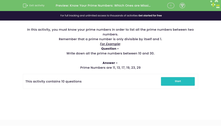### Comprehensive & curriculum aligned

In this worksheet, students will identify all the prime numbers between two given numbers.Key stage:  KS 2

Curriculum topic:   Number: Addition, Subtraction, Multiplication and Division

Curriculum subtopic:   Identify Common Factors, Multiples and Prime Numbers

Popular topics:   Numbers worksheets, Prime Numbers worksheets

Difficulty level:#### Worksheet Overview

In this activity, we will be testing our knowledge of prime numbers in order to list all the prime numbers between two numbers.

Remember that a prime number is only divisible by itself and 1. It has exactly two factors.Example
Write down all the prime numbers between 10 and 30.

Prime numbers have only two factors - the number 1 and the number itself.

We can immediately remove all the even numbers as possible prime numbers because they all have the number 2 as a factor.

So we can remove 12, 14, 16, 18, 20, 22, 24, 26 and 28.

That leaves us with 11, 13, 15, 17, 19, 21, 23, 25, 27 and 29 as possible contenders.

15 is not a prime number because it has 3 and 5 as its factors: 3 x 5 = 15

21 is not a prime number because it has 3 and 7 as its factors: 3 x 7 = 21

25 is not a prime number because it has 5 as a factor: 5 x 5 = 25

27 is not a prime number because it has 3 and 9 as its factors: 3 x 9 = 27

That leaves us with: 11, 13, 17, 19, 23 and 29 as the prime numbers between 10 and 30.

All of those numbers have only two factors.Let's have a go at some questions now.

### What is EdPlace?

We're your National Curriculum aligned online education content provider helping each child succeed in English, maths and science from year 1 to GCSE. With an EdPlace account you’ll be able to track and measure progress, helping each child achieve their best. We build confidence and attainment by personalising each child’s learning at a level that suits them.

Get started